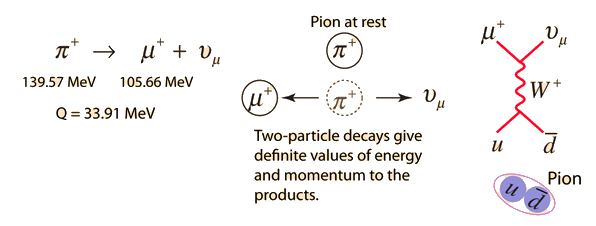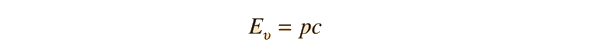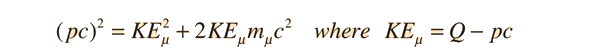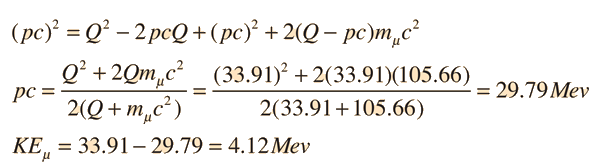# Energetics of Charged Pion Decay

Since the charged pions decay into two particles, a muon and a muon neutrino or antineutrino, then conservation of momentum and energy give the decay products definite energies. This contrasts with the three-particle decay of the neutral pion in which the emitted particles have a range of energies and momenta.The decay proceeds by the weak interaction and can be visualized in terms of Feynman diagrams. For the neutrino product which has negligible mass, the relativistic energy expression is justTo conserve momentum, the muon must have the same value of pc in the opposite direction. For the muon, we can write the momentum relationshipExpanding in terms of Q and pc, we obtainSeeing such definite energies identifies a decay as a two particle decay. The measurement of the kinetic energy of the muon at 4.13 MeV shows that most of the energy is carried by the neutrino, yet is consistent with zero mass for the neutrino since that value for the muon kinetic energy was calculated with the assumption of zero mass for the neutrino.

 Meson diagram Table of mesons
Index

Particle concepts

 HyperPhysics***** Quantum Physics R Nave
Go Back